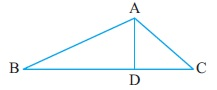Study Materials

# NCERT Solutions for Class 6th Mathematics

Page 4 of 6

## Chapter 4. Basic Geometrical Ideas

### Exercise 4.4

Exercise 4.4

Q1. Draw a rough sketch of a triangle ABC. Mark a point P in its interior and a point Q in its exterior. Is the point A in its exterior or in its interior?

Solution:

Q2. (a) Identify three triangles in the figure.
(b) Write the names of seven angles.
(c) Write the names of six line segments.
(d) Which two triangles have ∠B as common?Solution:

Page 4 of 6

Chapter Contents: